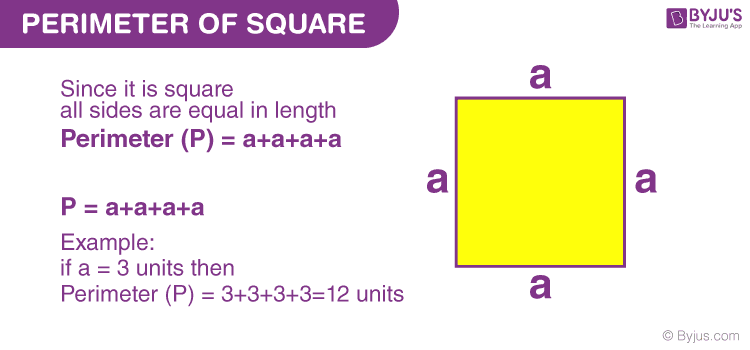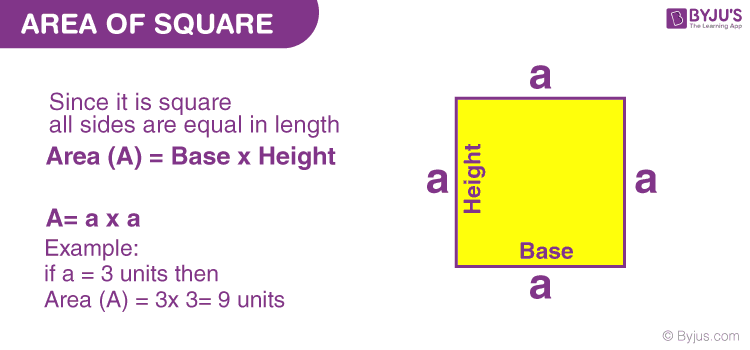# Perimeter of Square

The perimeter of a square is the length that its boundary covers. The perimeter of a square is obtained by adding all the sides together. Here, the concepts about the perimeter of the square, formula and derivation along with solved example questions are covered.

## What is the Perimeter of a Square?

The perimeter of any closed geometrical object is defined as the distance around that object. For a square, the perimeter is calculated by adding its four sides. We know that all the sides of a square are equal and hence, its perimeter will be 4 times its side, i.e. 4 × Side.

## The formula for Perimeter of a Square

 Perimeter of Square (P) = 4 × Side

### Derivation of a Square’s Perimeter

The perimeter of the square is defined as the length of the boundary of a square.

The Perimeter of the square formula is given by,

Perimeter = Sum of the lengths of 4 sides

= side + side + side + side

= 4 × side

Therefore, the perimeter of Square = 4s units

Where, s = Length of a side of the squareBelow is a brief recall about a square and its area.

### What is Square?

A square is a quadrilateral in which it has 4 equal sides and all the interior angles of a square are right angles. It is a closed two-dimensional plane figure.

## Area of Square

The area of a square is defined as the space occupied by the two-dimensional figure called square and the area of the square formula is given as

Area = width × height

Since width and height are the same, the formula is usually written as:

Area of a Square = Side × side = side² square units

where “s” is the length of one side.### Does Square have Surface Area?

People sometimes confuse the area of a square with the surface area. The surface area is, however for three dimensional figures such as a cube where there is more than one surface are involved and not for the planar shapes such as a square, which has only one surface.

Thus, to calculate the surface area of the square, one must follow the same method as to compute the area of the square.

### Solved Problems Using Perimeter of Square Concept

Question 1: Find the perimeter of a square whose side is 5 cm.

Solution:

Given:

Side, s = 5 cm

The formula to find the perimeter of a square is given by:

The perimeter of Square = 4s units

Substitute the value of ‘s’ in the perimeter formula,

P= 4 × 5 cm

P = 20 cm

Therefore, the perimeter of square = 20 cm

Question 2: Calculate the perimeter of a square having a side of 16 cm.

Solution:

Given,
Side of the square = a = 16 cm

Perimeter of a Square = 4a
= 4 × 16
= 64 cm

Question 3: If the perimeter of a square is 92 cm, find its side.

Solution:

Perimeter = 4 × side

Or, Side of Square = ¼ × perimeter

So, side of the square = 92/4 = 23 cm

### What is the Perimeter and Area of a Square?

The perimeter of a square is defined as the total distance around the outside of the square. So, it can be said that a square’s perimeter is its total boundary.

The area of a square is the region that the square covers in a two-dimensional space. The area of a square can also be defined as the number of square units it takes to fill the square.

### How to Find the Perimeter of a Square?

To calculate the perimeter of a square, add all the sides of it. There are 4 sides of a square which are all equal in length. The sum of the sides will give its perimeter.

### What is the Formula for the Perimeter of a Square?

The formula for the perimeter of a square is, P = Side + Side + Side + Side

Or, P = 4 × Side

Test your Knowledge on Perimeter of Square

1. Kagiso

This app helped me

2. edna howard

what is the area and perimeter of a square 10cm and 6cm?

1. Area of square (with side-length 10 cm) = 10^2 = 100 sq.cm.
Perimeter of square (with side-length 10 cm) = 4 x 10 = 40 cm

Area of square (with side-length 6cm) = 6^2 = 36 sq.cm.
Perimeter of square (with side-length 6cm) = 4 x 6 = 24 cm

3. Teacher you have help me

4. Thanks for doing my help